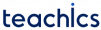# Depth First Search (DFS)

In this tutorial, you will learn the depth first search (DFS) algorithm for traversing a graph data structure with examples.

Depth-first search or depth-first traversal is a recursive algorithm used to visit all the vertices in a graph.

#### Depth First Search Algorithm

In the DFS algorithm, the traversal starts from a node and then follows its outgoing nodes. The search goes deeper and deeper until the goal node is found, or until a node that has no children is found.

DFS implementation puts each vertex in the graph into the following lists:

• Visited.
• Not Visited (Stack).

This marking is used to avoid cycles while traversing. The DFS algorithm performs the following operations:

1. Start from any vertex. PUSH it onto the stack.
2. POP the top item from the stack and add to the list of visisted nodes.
3. Select a adjacent vertex that is not visisted and PUSH it onto the stack.
4. Repeat steps 2 and 3 until stack is empty.

The formal algorithm is as follows:

```1: SET STATUS=1 (Not Visted) for each node in G
2: Push the starting node A on the stack and set its STATUS=2 (waiting state)
3: Repeat Steps 4 and 5 until STACK is empty
4: Pop the top node N. Process it and set its STATUS=3 (Visited)
5: Push on the stack all the neighbours of N that are not visisted (whose STATUS=1) and set their STATUS=2 (waiting state)
6: EXIT```

#### Depth First Search Algorithm Example

Consider the following graph with 5 vertices.

We start from vertex A. PUSH A onto the visited list and PUSH all its adjacent nodes(D, C, B) onto the stack.

Now POP D from the stack and visit it. Now visit the unvisited adjacent node(C) of D.

PUSH unvisited adjacent node C of vertex D onto the stack and visit it.

PUSH unvisited adjacent node E of vertex C onto the stack and visit it.

Finally, POP B from the stack and visit it.

#### Applications of Depth First Search Algorithm

• To Find a path between two specified nodes, u and v, in an unweighted or weighted graph.
• To determin whether a graph is connected or not.
• To calculate the spanning tree of a connected graph.
Subscribe
Notify of